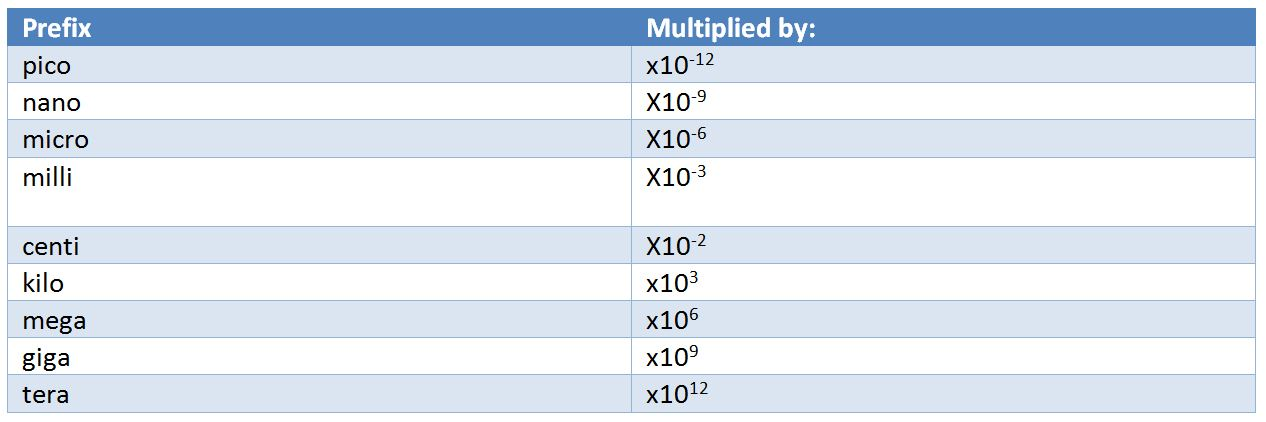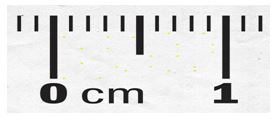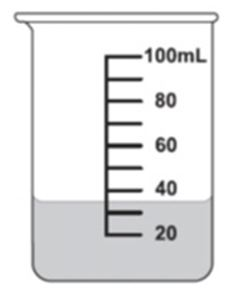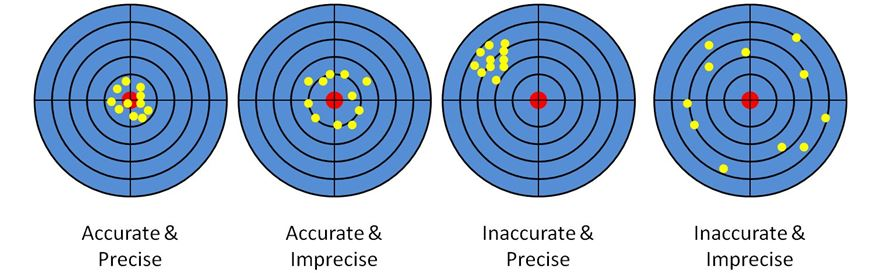# Physics Math Review## Units

It is very important in physics to use a standard set of units in all calculations so that we can discuss meaningful results. Predictions from theories or experimental results would make no sense unless uniform units are used throughout the calculations. Magnitudes of measurement can be compared much more easily if units are known. Density of materials, brightness of light, loudness of sound or length of a string; everything must have specific units of measurement.

There various sets of units such as MKS (meter-kilogram-seconds) units or CGS (centimeter-gram-seconds) units.

In physics, it is conventionally accepted to use S.I.units (Système International d’Unités).

## S.I. units

S.I.units is based on MKS units system. It is also known as the Metric system.

There are seven fundamental units in the SI system and all other units are derived from various combinations of these fundamental units.

The SI system of units is based on powers of 10. We use specific prefixes to denote powers of 10 (Eg Kilo, centi, milli, etc.)

i. The 7 fundamental units

• Meter – Meter is the unit of length, distance or displacement. It is officially defined as the distance travelled by light in vacuum in 1/299792458th of a second.

Sometimes when a meter is too small or large, units such as kilometers or centimeters are used rexpectively. The prefix ‘Kilo’ and ‘Centi’ will be explained in the next section.

• Kilogram – kilogram is the unit for mass. (be careful, kilogram is not a unit for weight. Weight is the force experienced by a mass due to the gravitational pull of a planet. )
• Seconds – seconds is the unit for time. 60seconds = 1minute and 60minutes = 1hour.
• Ampere – Ampere is the unit for measuring electric current.
• Candela – candela is the unit for measuring luminous intensity or in simpler words the brightness of light.
• Kelvin – this is the unit for measuring thermodynamic temperature. Although Celsius and Fahrenheit are more commonly used, in physics we use kelvin.
• Mole – mole is the measurement of amount of substance in numbers. It measures the number of elementary entities of a substance.

ii. Derived units

Lot of other different measurement are required to be made in physics. The units for such measurements can be derived from different combinations of the fundamental units. For eg:

• Velocity: velocity is displacement per unit time. So the unit is meter per second (m/s)
• Acceleration – acceleration is change is velocity per unit time. So the unit is meter per second per second or meter per second squared. So (m/s)/s I.e. m/s²
• Force – the unit of force is Newton. 1 Newton = 1 Kg × 1 m/s²

## Prefixes (powers of 10):

Sometimes these units are too small or too large. For Eg. When meter is too small we use kilometer. Instead of saying 6000m (or 6 × 10³ m) we say 6 Km. Thus we see the prefix Kilo used to denote an increase by a factor of 1000 or 10³

Similarly the prefix centi is used to denote division by a factor of 10² . So 6 cm = 6 × 10-2 m

Following are the prefix that are commonly used to denote multiplication by powers of 10.## Conversion of units

i. Conversion of fundamental units with different prefix

• Say we want to convert a 6,230 m to Km. From the table of prefix we know that 1 Km = 1 × 10³ m.

If we divide both sides by 10³ we get,

(1/10³) Km = (1 × 10³/10³) m

So, 10-3 Km = 1 m

If, 1 m = 10-3 Km

Then, 6,230 m = 6,230 × 10-3 km=6.230 Km

• We can also use another method where we multiply the desired length (in meters) to the ratio 1Km/1000m (Since 1000m=1Km, this ratio is actually 1 and hence doesn’t increase or decrease the length) This will cancel out the unit meters and convert the length in Km. This method is explained in detail in the lecture AP Physics 1 & 2: Math Review.
• Although conversion seems easy, one should follow these steps and be careful so as to not make mistakes during calculations.

ii. Conversion of derived units

• When we are converting derived units, we need to convert all the concerned units separately. We will use an example to familiarize with it.
• Eg: convert 20m/s to km/hr. We need to convert meter to Km in numerator and seconds to hour in denominator.

So 20 m = 20 × 10-3Km [similar to the previous example].

Now 1 hr=60 min

And 1 min = 60 sec

So 1 hr = 60 min = 60 x 60 sec = 3600 sec

So 1 sec = (1/3600) hr [dividing both sides of the above equation by 3600]

20 m/s = 20 x 10-3 Km/(1/3600) hr

So, 20 m/s = 0.02 x 3600 Km/hr = 72 Km/hr

## Significant Figures

Significant figures are those digits in any measurement or calculations which are known with some level of certainty. The number of significant digits in any measurement show the exactness of the results.

i. Measuring with significant figures

To know the number of significant figures in our measurement data we need to proceed as follows:

• Write down as many digits you can from the measurement with absolute certainty. For eg. If you have a scale with markings for every cm, then you can measure the length to accurately upto a cm.Or as we can see in this picture there are 10 markings for every cm so we can measure with certainty of 0.1cmThis beaker has markings for every 10ml. So we can measure with certainity for 10 ml, 20 ml, 30 ml or so on.

• Next we take a guess at reading one more digit.

For eg. in the beaker we know for certain there is 30ml. Next we take a guess, whether its 35ml or 36ml according to our best judgment.

This resulting value is the quantity in significant figures.

ii. Reading significant figures

We should keep in mind the following rules to read the number of significant digits in a measurement :

• All non-zero digits are significant
• All the digits between two non-zero digits are significant
• Zeroes to the right of the significant digits are significant
• Zeroes to the left of significant digits are not significant

Examples: Lets see how many significant digits the following numbers have:

• 3200 Kg

So 3 and 2 are non-zero digits and hence significant. There are two zeroes on the right of significant digits and hence are also significant. Thus there are four significant digits.

• 0.302 m

3 and 2 are non zero and hence significant. There is a zero in between the two significant digits and hence is also significant. But there is a zero to the left of three, so this zero is not significant. Thus there are only three significant digits.

• 4.0906

4,9 and 6 being non-zero are significant. There are two zeroes and both of them are in between two significant figures. Thus both zeroes are significant. So we have five significant digits in this number.

## Scientific notation

Scientific notation (also referred to as standard form or standard index form) is a way of expressing numbers that are too big or too small to be conveniently written in decimal form.

i. Why scientific notation

Measurements vary largely in magnitude. The length of a desk could be just a meter, the radius of string could be 0.1mm while the distance between two towns could be in hundreds of Km.

If we were to express all of them in them in similar units, it would become very inconvenient unless we use scientific notations.

Eg. 4.20 x 10-6 m is much easier to comprehend than 0.00000420 m

ii. How to write scientific notations

To write down measurements in scientific notations follow these steps:

• Show your measurement value in correct number of significant figures.
•  Move your decimal point so that only one significant digit is to the left of the decimal point
• Multiply this number with the appropriate power of 10.

iii. Addition/Subtraction

Before adding or subtracting numbers written in scientific notation, make sure they are converted to the same powers of 10.

iv. Exercise

• Write in scientific notation: 0.000782

Ans: 7.82 × 10-4

• Express 5.62 x 105 as a number

Ans: 562000

• Add 2.98 × 104 + 9.1 × 107

Ans: Convert 2.98 × 104 to power of 7.

So it becomes 0.00298 × 107.

Now add 0.00298 × 107 +9.1 × 107 = 9.10298 × 107

## Accuracy and precision

i. Accuracy

Accuracy refers to the closeness of a measured value to a standard or known value

ii. Precision

Precision refers to the closeness of two or more measurements to each other

A helpful way to understand the difference is with example of error while shooting at a target• If all the shots hit the intended target then its both accurate and precise
• If none of the shots hit the same spot, then they are not precise. But If they all hit very close to the target then they are still accurate,
• If they all hit quite far away from the target, but all the shots hit almost the same spot, then they are not accurate but they are precise.
• If they hit far away from intended target and are scattered away from each other, then they are neither accurate nor precise.

Accuracy and precision are independent of each other. Accuracy is associated with observational error while precision is associated with random error.

For more information check out our AP Physics 1 & 2 course more topics such as Math Review and Friction.